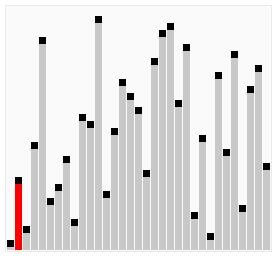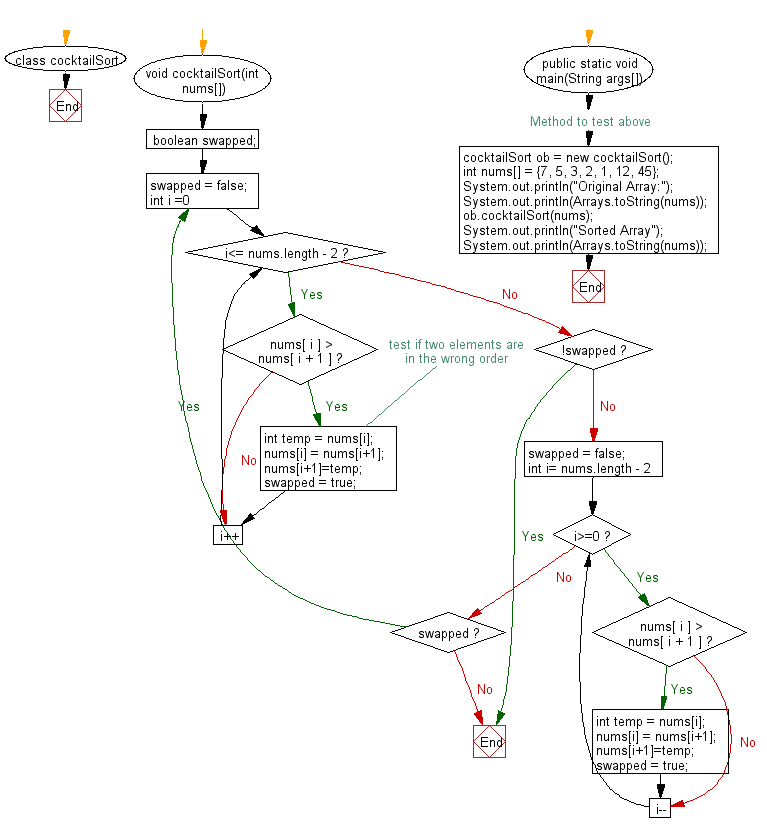﻿ Java exercises: Cocktail sort Algorithm - w3resource# Java Exercises: Cocktail sort Algorithm

## Java Sorting Algorithm: Exercise-10 with Solution

Write a Java program to sort an array of positive integers using the Cocktail sort Algorithm.

Cocktail shaker sort (also known as bidirectional bubble sort, cocktail sort, shaker sort, ripple sort, shuffle sort, or shuttle sort ) is a variation of bubble sort that is both a stable sorting algorithm and a comparison sort. The algorithm differs from a bubble sort in that it sorts in both directions on each pass through the list. This sorting algorithm is only marginally more difficult to implement than a bubble sort and solves the problem of turtles in bubble sorts. It provides only marginal performance improvements, and does not improve asymptotic performance; like the bubble sort, it is not of practical interest, though it finds some use in education.

Visualization of shaker sort :Sample Solution:

Java Code:

``````import java.util.Arrays;
class cocktailSort
{
void cocktailSort(int nums[])
{
boolean swapped;
do {
swapped = false;
for (int i =0; i<=  nums.length  - 2;i++) {
if (nums[ i ] > nums[ i + 1 ]) {
//test if two elements are in the wrong order
int temp = nums[i];
nums[i] = nums[i+1];
nums[i+1]=temp;
swapped = true;
}
}
if (!swapped) {
break;
}
swapped = false;
for (int i= nums.length - 2;i>=0;i--) {
if (nums[ i ] > nums[ i + 1 ]) {
int temp = nums[i];
nums[i] = nums[i+1];
nums[i+1]=temp;
swapped = true;
}
}
} while (swapped);
}
// Method to test above
public static void main(String args[])
{
cocktailSort ob = new cocktailSort();
int nums[] = {7, 5, 3, 2, 1, 12, 45};
System.out.println("Original Array:");
System.out.println(Arrays.toString(nums));
ob.cocktailSort(nums);
System.out.println("Sorted Array");
System.out.println(Arrays.toString(nums));
}
}
```
```

Sample Output:

```Original Array:
[7, 5, 3, 2, 1, 12, 45]
Sorted Array
[1, 2, 3, 5, 7, 12, 45]
```

Flowchart:Java Code Editor:

What is the difficulty level of this exercise?

﻿

## Java: Tips of the Day

Array vs ArrayLists:

The main difference between these two is that an Array is of fixed size so once you have created an Array you cannot change it but the ArrayList is not of fixed size. You can create instances of ArrayLists without specifying its size. So if you create such instances of an ArrayList without specifying its size Java will create an instance of an ArrayList of default size.

Once an ArrayList is full it re-sizes itself. In fact, an ArrayList is internally supported by an array. So when an ArrayList is resized it will slow down its performance a bit as the contents of the old Array must be copied to a new Array.

At the same time, it's compulsory to specify the size of an Array directly or indirectly while creating it. And also Arrays can store both primitives and objects while ArrayLists only can store objects.

Ref: https://bit.ly/3o8L2KH